##### The Japanese Bonsai specialist
Direct order Contact Help / Services Newsletter Pay in 3 times without charges, from 135 euros in your shopping cart (France residents only)# Ginkgo biloba

› Young maples and bonsai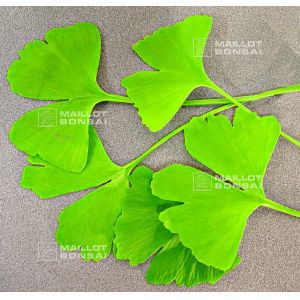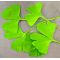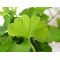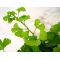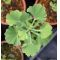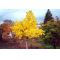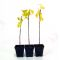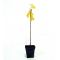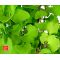ref. : 1180

5,40

Available quantity : 2Order

###### Description

Quick green foliage of green spring darkened then in summer pass by colorations of yellow fall of gold. Species marks. Delivered in plastic pot. Height to the delivery + - 40 cm.

Description :
Scion descended of seedling of + -40 cm in second year of culture.
Ø to the collar + - 5/7 mm according to topics.

The plants descended of seedling can present disparities of shapes and colors of leaves.

#descended 2.9 #seedling 2.8 #bonsai 2.6 #maples 2.6 #biloba 2.6 #ginkgo 2.6 #young 2.5 #green 2.5 #description 2.1 #colorations 2.1

Formule
(( ROUND((CHAR_LENGTH(b.article_nom)-CHAR_LENGTH(REPLACE(b.article_nom, 'descended', '')))/LENGTH('descended')) + ROUND((CHAR_LENGTH(b.article_description)-CHAR_LENGTH(REPLACE(b.article_description, 'descended', '')))/LENGTH('descended')) ) * 2.9) + (( ROUND((CHAR_LENGTH(b.article_nom)-CHAR_LENGTH(REPLACE(b.article_nom, 'seedling', '')))/LENGTH('seedling')) + ROUND((CHAR_LENGTH(b.article_description)-CHAR_LENGTH(REPLACE(b.article_description, 'seedling', '')))/LENGTH('seedling')) ) * 2.8) + (( ROUND((CHAR_LENGTH(b.article_nom)-CHAR_LENGTH(REPLACE(b.article_nom, 'biloba', '')))/LENGTH('biloba')) + ROUND((CHAR_LENGTH(b.article_description)-CHAR_LENGTH(REPLACE(b.article_description, 'biloba', '')))/LENGTH('biloba')) ) * 2.6) + (( ROUND((CHAR_LENGTH(b.article_nom)-CHAR_LENGTH(REPLACE(b.article_nom, 'ginkgo', '')))/LENGTH('ginkgo')) + ROUND((CHAR_LENGTH(b.article_description)-CHAR_LENGTH(REPLACE(b.article_description, 'ginkgo', '')))/LENGTH('ginkgo')) ) * 2.6) + (( ROUND((CHAR_LENGTH(b.article_nom)-CHAR_LENGTH(REPLACE(b.article_nom, 'green', '')))/LENGTH('green')) + ROUND((CHAR_LENGTH(b.article_description)-CHAR_LENGTH(REPLACE(b.article_description, 'green', '')))/LENGTH('green')) ) * 2.5) + (( ROUND((CHAR_LENGTH(b.article_nom)-CHAR_LENGTH(REPLACE(b.article_nom, 'disparities', '')))/LENGTH('disparities')) + ROUND((CHAR_LENGTH(b.article_description)-CHAR_LENGTH(REPLACE(b.article_description, 'disparities', '')))/LENGTH('disparities')) ) * 2.1) + (( ROUND((CHAR_LENGTH(b.article_nom)-CHAR_LENGTH(REPLACE(b.article_nom, 'colorations', '')))/LENGTH('colorations')) + ROUND((CHAR_LENGTH(b.article_description)-CHAR_LENGTH(REPLACE(b.article_description, 'colorations', '')))/LENGTH('colorations')) ) * 2.1) + (( ROUND((CHAR_LENGTH(b.article_nom)-CHAR_LENGTH(REPLACE(b.article_nom, 'description', '')))/LENGTH('description')) + ROUND((CHAR_LENGTH(b.article_description)-CHAR_LENGTH(REPLACE(b.article_description, 'description', '')))/LENGTH('description')) ) * 2.1) + (( ROUND((CHAR_LENGTH(b.article_nom)-CHAR_LENGTH(REPLACE(b.article_nom, 'according', '')))/LENGTH('according')) + ROUND((CHAR_LENGTH(b.article_description)-CHAR_LENGTH(REPLACE(b.article_description, 'according', '')))/LENGTH('according')) ) * 1.9) + (( ROUND((CHAR_LENGTH(b.article_nom)-CHAR_LENGTH(REPLACE(b.article_nom, 'delivered', '')))/LENGTH('delivered')) + ROUND((CHAR_LENGTH(b.article_description)-CHAR_LENGTH(REPLACE(b.article_description, 'delivered', '')))/LENGTH('delivered')) ) * 1.9)

## Secure payment## Delivery

Our logistic partners :